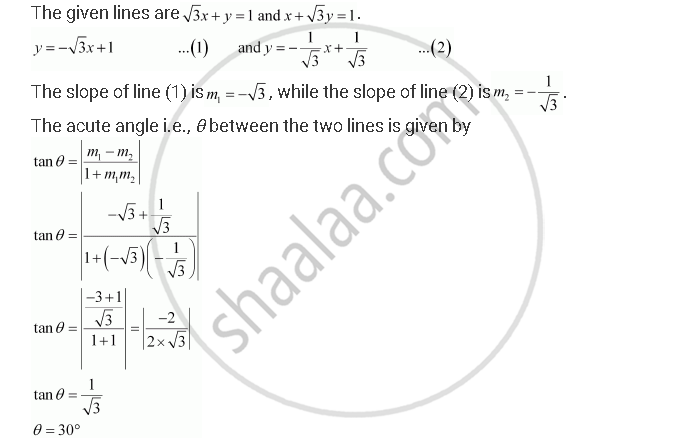CBSE (Arts) Class 11CBSE
Share

# Find Angles Between the Lines Sqrt3x + Y = 1 And X + Sqrt3y = 1 - CBSE (Arts) Class 11 - Mathematics

ConceptGeneral Equation of a Line

#### Question

Find angles between the lines sqrt3x + y = 1 and  x +     sqrt3y = 1

#### SolutionThus, the angle between the given lines is either 30° or 180° – 30° = 150°.

Is there an error in this question or solution?

#### Video TutorialsVIEW ALL 

Solution Find Angles Between the Lines Sqrt3x + Y = 1 And X + Sqrt3y = 1 Concept: General Equation of a Line.
S